## Deep Learning with Keras and TensorFlow

Certification Training
8490 Learners
7 Chapters +

# Introduction to TensorFlow

Welcome to the fifth lesson ‘Introduction to TensorFlow’ of the Deep Learning Tutorial, which is a part of the Deep Learning (with TensorFlow) Certification Course offered by Simplilearn. This lesson introduces you to the concept of TensorFlow.

Let us begin with the objectives of this lesson.

## Objectives

After completing this lesson on Multilayer ANN, you’ll be able to:

• Explore the meaning of TensorFlow

• Create a computational and default graph in TensorFlow

• Demonstrate reshaping of a tensor with tf.reshape

• Implement Linear Regression and Gradient Descent in TensorFlow

• Discuss the meaning and application of Layers and Keras in TensorFlow

• Demonstrate the use of TensorBoard

In the next section, let us learn about TensorFlow.

## What is TensorFlow?

TensorFlow can be defined as follows:

• A popular Machine Learning library, particularly well suited for large-scale Machine Learning

• An open source software library for numerical computation using data flow or computational graphs

• A distributed computing tool that enables colossus neural networks to be trained across distributed servers. Google has also launched a cloud service to run TensorFlow graphs.

In the next section, let us see TensorFlow at a glance.

Preparing to become an expert in Deep Learning? Consider enrolling in our Course now!

## TensorFlow At A Glance

TensorFlow has the following characteristics:

• It includes highly efficient C++ implementations of Machine Learning and custom C++ operations.

• High-level APIs like TF Layers, Keras and Pretty Tensor can run on top of TensorFlow. It provides simple API TF-Slim (tensorflow.contrib.slim) for simple training routines.

• It runs on Operating Systems like Windows, Linux, and macOS, and mobile operating systems like iOS and Android.

• It provides several optimization nodes to search for parameters that minimize a cost function.

• It automatically computes gradients of the cost functions. This is called automatic differentiating (Autodiff).

• It provides a visualization tool called TensorBoard, in which one can view the computation graph, learning curves etc.

• It provides a simple Python API called TF.Learn (tensorflow.contrib.learn) for training neural networks in few lines of code.

In the next section, let us focus on the types of APIs that the TensorFlow provides.

## Types of APIs

Two Main APIs that TensorFlow provides are:

1. TensorFlow Core API - Low-level Machine Learning development

2. Higher Level APIs - More compact APIs such as tf.contrib.learn, or tf.layers

TensorFlow Core API

TensorFlow Core provides complete programming control. It is more suitable for machine learning researchers and others who require fine levels of control over their models.

Higher level APIs

Higher level APIs are built on top of TensorFlow Core. These are easier to learn and use than TensorFlow Core. They make repetitive tasks easier and more consistent between different users.

Higher level API such as tf.contrib.learn helps you manage data sets, estimators, training and inference.

In the next section, let us learn what the Tensors are.

## What are Tensors?

Tensors are multidimensional data arrays or inputs and outputs of TensorFlow. They are a set of values shaped into an n-dimensional array with static and dynamic type dimensions. They mathematically represent a physical entity, characterized by magnitude and multiple directions.

They typically contain floats but can carry strings too in the form of byte arrays. They may be passed between the nodes of a computation graphs and have a data type.

NumPy is a Python API used for numerical computations.

Let us learn about Tensor Ranks in the next section.

## Tensor Ranks

A tensor’s dimensionality can be described using rank, shape and dimension number. Tensor’s shape and dimensions determine its rank. The rank of a tf.tensor object is its number of dimensions.

Number of Dimensions + Shape determines Tensor Rank

Tensor Rank 0 - 3#a + Scalar shape with [ ]

Tensor Rank 1 - [1. ,2., 3.] # a + vector with shape 

Tensor Rank 2 - [[1., 2., 3.], [4., 5., 6.]] # a + a matrix with shape [2, 3]

Tensor Rank 3 - [[[1., 2., 3.]], [[7., 8., 9.]]] # a + with shape [2, 1, 3]

Let us look at how to install TensorFlow in the next section.

## How to Install TensorFlow

Install TensorFlow:

- \$ pip3 install  -upgrade tensorflow

For GPU installation of TF:

- \$ pip3 install –upgrade tensorflow-gpu

Test the installation:

- \$ python3 -c 'import tensorflow; print(tensorflow.__version__)‘

- 1.0.0

## Open Source Deep Learning Libraries

There are other libraries that perform deep learning with almost similar capabilities. However, Google’s TensorFlow has proven to be a scalable, clean, flexible, and efficient library.

The reason that it is backed by Google, has caused it to catapult to the top of the developer’s choice.

In the next section, let us learn about Computational Graphs.

## Computational Graph

TensorFlow operations can be arranged into a graph termed as a computational graph.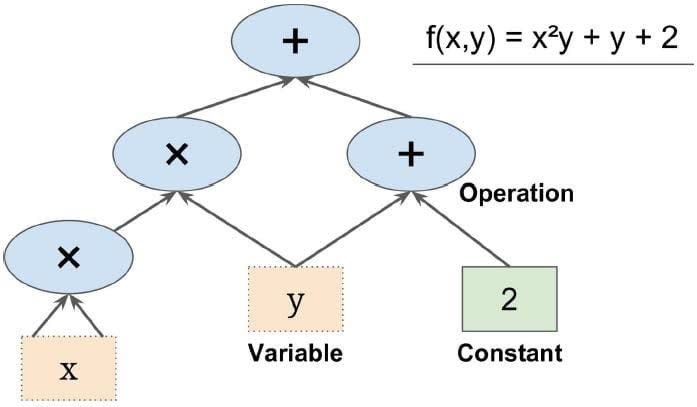It enables creating very large scale neural networks as computing can be distributed across several CPUs or GPUs in a similar fashion.

Computational Graph is the graph of program logic which TensorFlow builds in the memory.

It demonstrates how variables are defined and operations are performed on them to solve the desired function.

The actual execution of the graph comes in the steps that follow later.

The computational graph can be broken into chunks and can run across many different CPUs or GPUs parallelly. This is called parallel computation.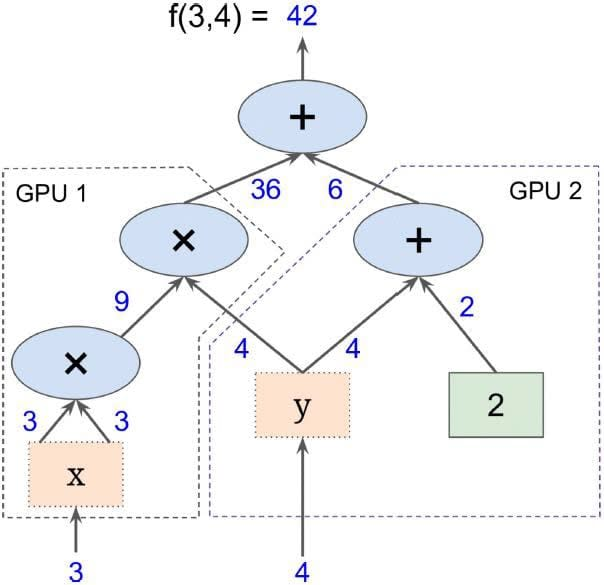Let us focus on the program elements of TensorFlow in the next section.

## TensorFlow Program Elements

The TensorFlow program elements are discussed one by one below:

Constant

A parameter whose value never changes

Placeholder

Permits a value to be assigned later

a = tf.placeholder(tf.float32)

feed_dict parameter

Specifies Tensors that provide concrete values to the placeholders

Variable

Allows addition of new trainable parameters to a graph

W = tf.Variable([.3], dtype=tf.float32)

b = tf.Variable([-.3], dtype=tf.float32)

x = tf.placeholder(tf.float32)

linear_model = W * x + b

Session

A session is run to evaluate the nodes

This is called as the TensorFlow runtime

## Creating Computational Graph

Let us learn how to create computational graph in the following topics.

CONSTRUCTION PHASE

The code given here creates a graph in memory.

import tensorflow as tf

x = tf.variable(3, name=”x”)

y = tf.variable(4, name=”y”)

f = x*x*y + y + 2

It declares variables and function.

This creates a TF graph in memory corresponding to the objects created by the code (the actual execution of this logic does not happen yet).

EXECUTION PHASE

>>> sess = tf.Session( )

>>> sess.run( x.initializer )

>>> sess.run( y.initializer )

>>> result = sess.run( f )

>>> print( result )

42

>>> sess.close

To evaluate the graph, open TensorFlow session and run it.

The above set of code runs a TF session to execute the three graph nodes (one each for x, y, and f) and print the result of the entire operation.

With tf.Session( ) as sess:

x.initializer.run( )

y.initializer.run( )

result = f.eval( )

To avoid calling sess.run multiple times; you can run the above code.

The above-given set of code encapsulates the running of three graph nodes under one session run.

INITIALIZATION PHASE

In the previous code, you initialized x and y separately.

In the given code, you initialize it in one shot using a global initializer.

init = tf.global_variables_initializer( )    # prepare an init node

with tf.Session( ) as sess:

init.run( )   # actually initialize all the variables

result = f.eval( )

So, instead of initializing each variable separately, global_variables_initializer can be used to initialize all the variables together:

INITIALIZATION AND EXECUTION PHASE

>>> sess = tf.InteractiveSession( )

>>> init.run( )

>>> result = f.eval( )

>>> print(result)

42

>>> sess.close( )

The above code removes the need to encapsulate the session execution in a "with" block.

An InteractiveSession(instead of Session) sets up a default session, therefore, a “with”block is no longer required.

In the next section, let us learn about default graphs.

## Default Graph

In Jupyter (or in a Python shell), it is common to run the same commands more than once while you are experimenting. As a result, you may end up with a default graph containing many duplicate nodes.

One solution is to restart the Jupyter kernel (or the Python shell), but a more convenient solution is to just reset the default graph by running tf.reset_default_graph().

For example, if you have a line of code x = tf.variable (3, name="x") and you run the program more than once or twice within the same Python shell, it would create multiple duplicate copies of the node pertaining to the variable x.

This can be avoided by a number of techniques like resetting a graph.

The default graph is the graph that is produced by default for a TensorFlow program.

>>> x1 = tf.Variable(1)

>>> x1.graph is tf.get_default_graph( )

True

You can create other graphs programmatically (apart from the default graph) in certain situations if required.

Any new node gets added to default graph, unless the newly created graph is set as the default graph, for example, the case of variable x2 as shown in the next set of code.

>>> graph = tf.Graph( )

>>> with graph.as_default( ):

. . .   x2 = tf.Variable(2)

. . .

>>> x2.graph is graph

True

>>> x2.graph is tf.get_default_graph( )

False

To use a new graph, create a Graph and use a “with” block to temporarily make it the default graph.

In the next section, let us focus on the lifecycle of a node value.

## The Lifecycle of a Node Value

A Node refers to an object in the computation graph. It can be a variable or constant or an operation.

W = tf.constant(3)

x = w + 2

y = x + 5

z = x + 3

With tf.Session( ) as sess:

print (y.eval())     # 10

print (z.eval())     # 15

Y is evaluated after the session starts, but it depends on x, which in turn depends on w.

So computation order is w, x, and then y.

with tf.Session( ) as sess

y_val, z_val = sess.run ( [y, z])

print(y_val)   # 10

print(z_val)   # 15

When z is evaluated, it does not reuse computed value of x and w but re-computes them instead.

Variables, however, maintain their value between graph executions.

Y and z get computed in one graph run, thus avoiding two different computations for x and w.

Let us focus on the shape of the tensor in the next section.

## The Shape of a Tensor

TensorFlow provides several operations that you can use to determine the shape of a tensor and change the shape of a tensor.

The following code returns the shape of a tensor:

tf.shape(input, name=None, out_type=tf.int32)

The above statement can be explained as shown: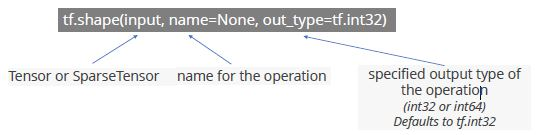It returns the shapes of a tensor using 1D integer tensor of type out_type.

In the next section, let us learn the size of a tensor.

## Size of a Tensor

The following code returns the size of a tensor:

tf.size(input, name=None, out_type=tf.int32)

The above statement can be explained as shown: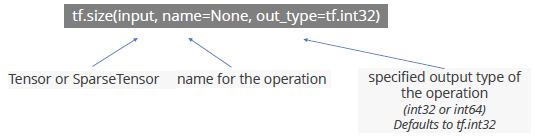It returns the size of a tensor representing the number of elements in the input.

Let us learn how to reshape a Tensor in the next section.

Willing to take up a course in Deep Learning? Check out our Course Preview now!

## Reshaping of a Tensor

The following code reshapes a tensor.

tf.reshape(tensor, shape, name=None)

The above statement can be explained as shown: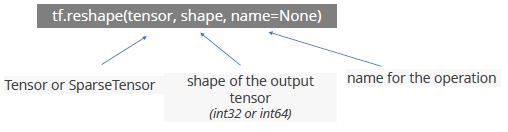If a tensor is given, this operation returns a tensor that has the same values as “tensor” with shape “shape.”

If one component of shape is the special value -1, the size of that dimension is computed so that the total size remains constant.

In particular, a shape of [-1] flattens into 1-D.

At most one component of shape can be -1.

Example 1

# tensor 't' is [1, 2, 3, 4, 5, 6, 7, 8, 9]

# tensor 't' has shape 

reshape(t, [3, 3]) ==> [[1, 2, 3],

[4, 5, 6],

[7, 8, 9]]

In the code given above:

• A tensor t is declared with shape .

• It is then reshaped to [3,3] shape and output is checked.

• Tensor t has shape [x, y, z] where,

x is no of rows

y is no of columns

z is depth

Example 2

# tensor 't' is [[[1, 1], [2, 2]],

# [[3, 3], [4, 4]]]

# tensor 't' has shape [2, 2, 2]reshape(t, [2, 4]) ==> [[1, 1, 2, 2],

[3, 3, 4, 4]]

In the code given above:

• A tensor "t" is declared with shape [2,2,2].

• It is then reshaped to [2,4] shape and output is checked.

Example 3

Create a tensor of shape [3,2,3] and flatten it to 1D with [-1] notation in the reshape command.

# tensor 't' is [[[1, 1, 1],

# [2, 2, 2]],

# [[3, 3, 3],

# [4, 4, 4]],

# [[5, 5, 5],

# [6, 6, 6]]]

# tensor 't' has shape [3, 2, 3]

# pass '[-1]' to flatten 't'

reshape(t, [-1]) ==> [1, 1, 1, 2, 2, 2, 3, 3, 3, 4, 4, 4, 5, 5, 5, 6, 6, 6]

# -1 can also be used to infer the shape

# -1 is inferred to be 9:

reshape(t, [2, -1]) ==> [[1, 1, 1, 2, 2, 2, 3, 3, 3],

[4, 4, 4, 5, 5, 5, 6, 6, 6]]

# -1 is inferred to be 2:

reshape(t, [-1, 9]) ==> [[1, 1, 1, 2, 2, 2, 3, 3, 3],

[4, 4, 4, 5, 5, 5, 6, 6, 6]]

# -1 is inferred to be 3:

reshape(t, [ 2, -1, 3]) ==> [[[1, 1, 1],

[2, 2, 2],

[3, 3, 3]],

[[4, 4, 4],

[5, 5, 5],

[6, 6, 6]]]

# tensor 't' is 

# shape `[]` reshapes to a scalar

reshape(t, []) ==> 7

In the next section, let us learn the Linear Regression.

## Linear Regression

Linear regression is a linear approach for modeling the relationship between a scalar dependent variable y and an independent variable x.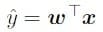x and y are vectors of real numbers and w is a vector of weight parameters.

The equation is also written as:

y= wx + b

where b is the bias or the value of output for zero input.

The graph depicting linear regression is as shown: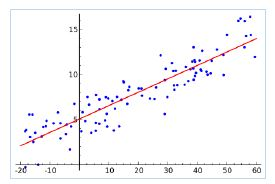Linear Regression can be of two types:

• Simple Linear Regression

• Multiple Linear Regression

In the next section, let us learn the Gradient Descent.

Gradient descent is an optimization algorithm used for finding the weights or coefficients of machine learning algorithms.

The goal of the algorithm is to find model parameters like coefficients or weights that minimize the error of the model on the training dataset.

This is done by making changes to the model that move it along a gradient or slope of errors down toward a minimum error value. This gives the algorithm the name gradient descent.

The three types of gradient descent are:

• Batch

• Stochastic

• Mini-batch

Mini-batch gradient descent splits the training dataset into small batches that are used to calculate model error and update model coefficients.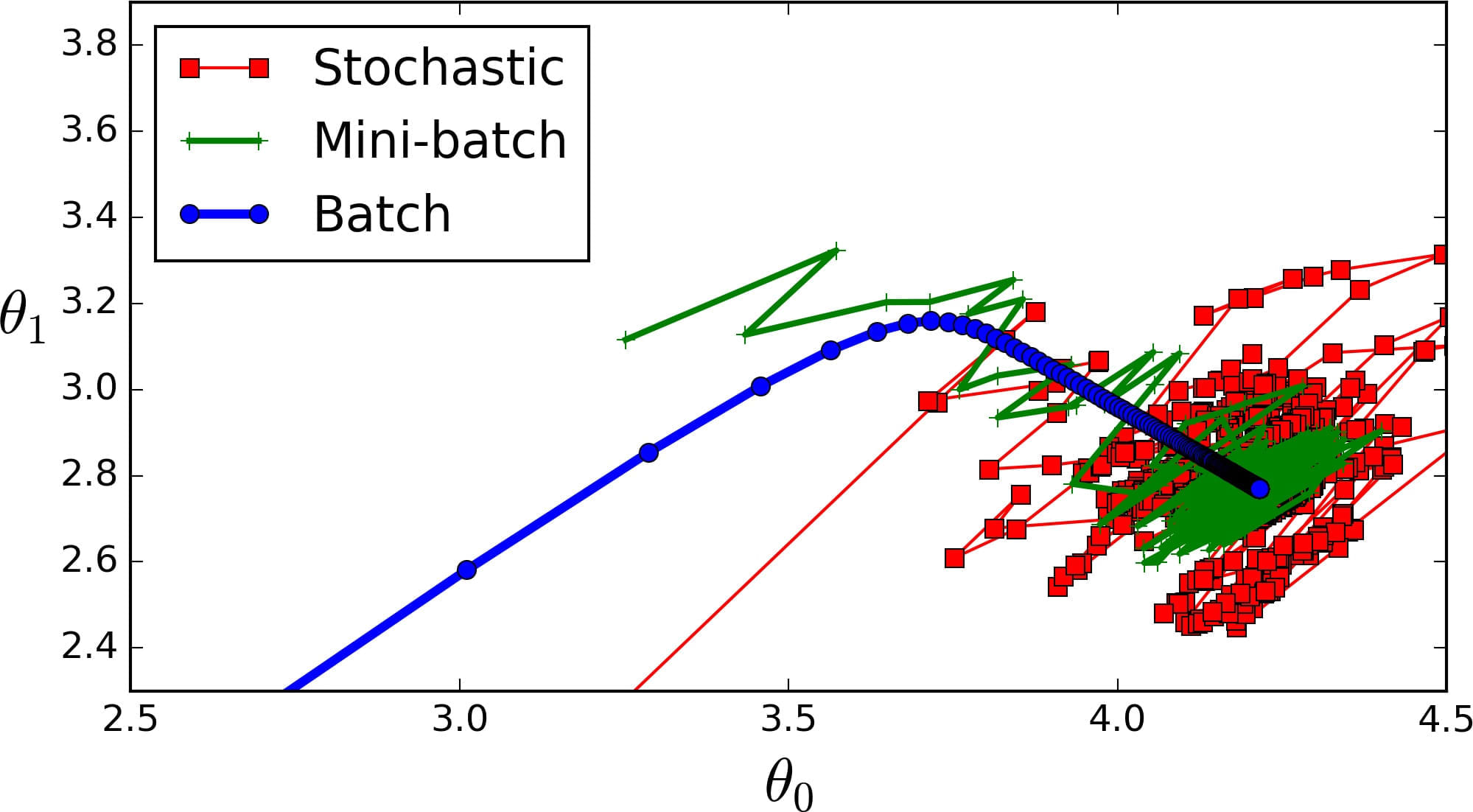In the next section, let us learn the linear regression in TensorFlow.

## Linear Regression in TensorFlow

In order to train a data model, TensorFlow loops through the data and finds the optimal line that fits the data. The linear relationship between two variables X and Yis estimated by designing an appropriate optimization problem.tf.estimator API in TensorFlow can be used to solve a binary classification problem

The following code is a Linear Regression model with TensorFlow, with a given a set of training data x_train and y_train.

Import numpy as np

Import tensorflow as tf

# Model parameters

W = tf.Variable([.3], tf.float32)

b = tf.Variable([-.3], tf.float32)

# Model input and output

x = tf.placeholder(tf.float32)

linear_model = W * x + b

y = tf.placeholder(tf.float32)

# loss

loss = tf.reduce_sum(tf.square(linear_model -y))

# sum of the squares

# optimizer

train = optimizer.minimize(loss)

# training data

x_train = [1,2,3,4]

y_train = [0,-1,-2,-3]

# training loop

init = tf.global_variables_initializer()

sess = tf.Session()

sess.run(init) # reset values to wrong

For i in range(1000):

sess.run(train, {x:x_train, y:y_train})

# evaluate training accuracy

curr_W, curr_b, curr_loss = sess.run([W, b, loss], {x:x_train, y:y_train})

print("W: %s b: %s loss: %s"%(curr_W, curr_b, curr_loss))

OUTPUT

In this model, you first declare placeholders for x, y, weights w and bias b.

Then use MSE error as cost function and minimize it using the Gradient Descent optimizer.

Run the session to find ideal weights and then print the training accuracy.

The output produced after the execution is as follows:

W: [-0.9999969]

b: [ 0.99999082]

loss: 5.69997e-11

The graph shows the fitted regression line after the model training.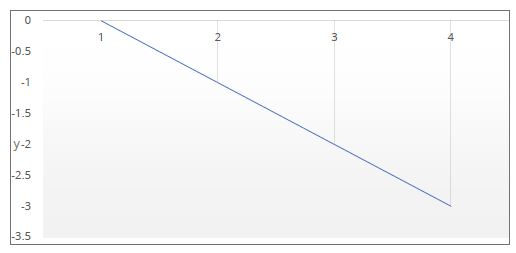Algorithm learned:

y = (-0.9999969) * x + 0.99999082

Let us study how to visualize using TensorBoard in the next section.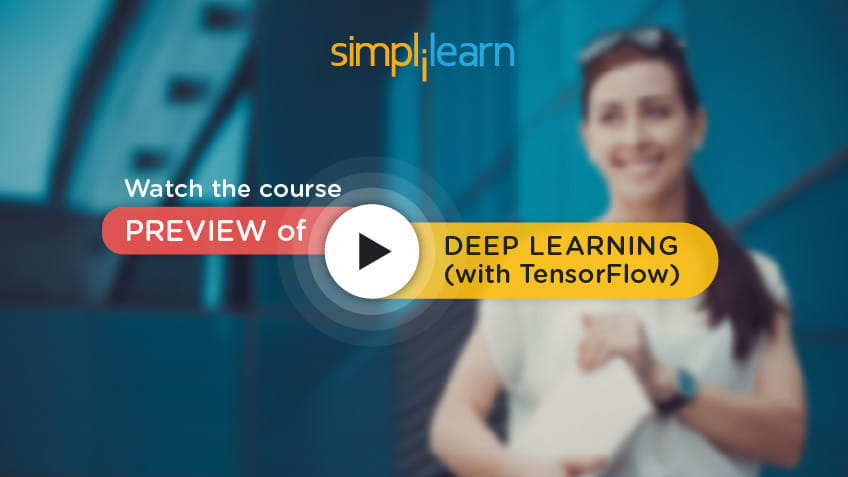## Visualization Using TensorBoard

The image shows the Computation Graph for Linear Regression code shown on previous slides.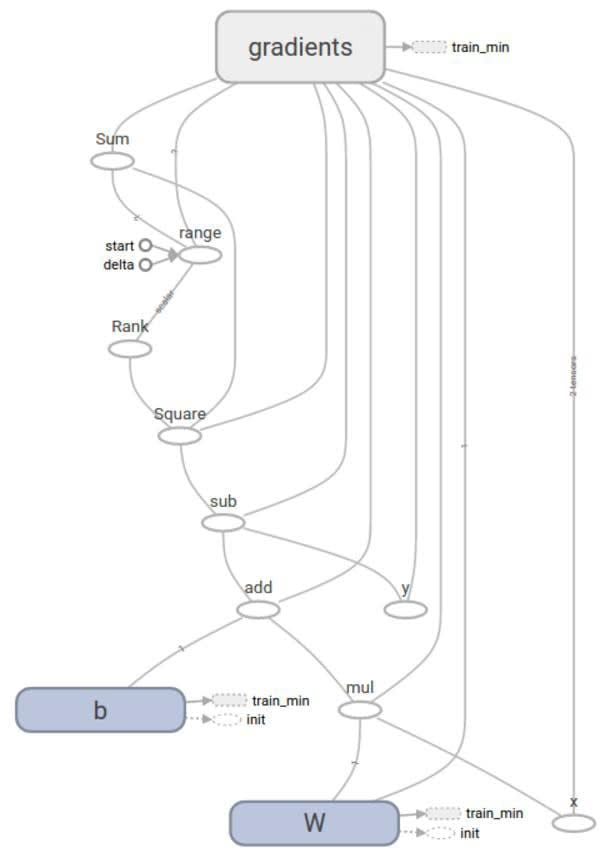The various nodes correspond to weights, biases etc, as well as various operations from that code.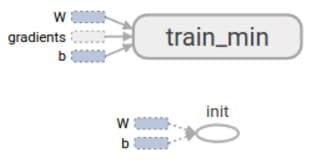Linear Regression for the California housing dataset using Normal Equation method

The code shows Linear Regression for the California housing dataset. Here weights are calculated using the Normal Equation:

θ = (XT.X)-1.XT.y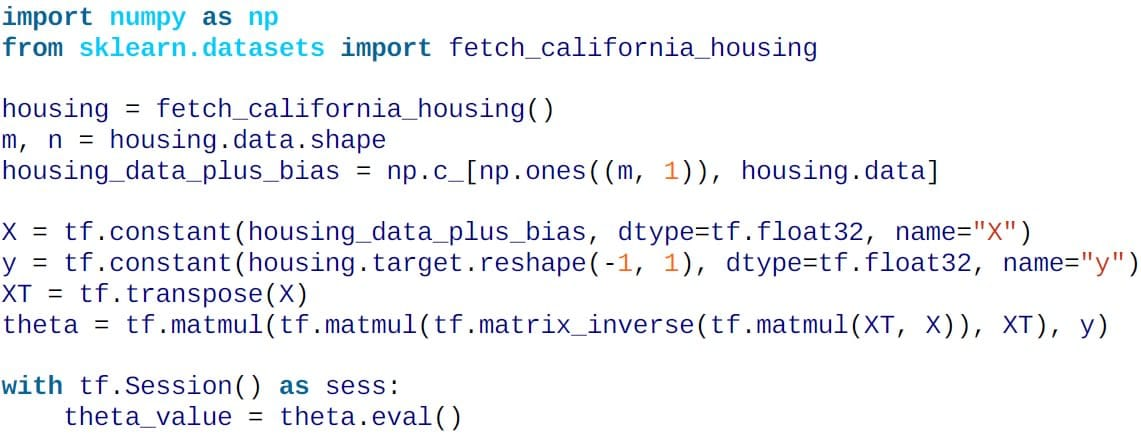One could have used normal NumPy to run the Normal Equation, but it would not run on GPU, whereas TensorFlow can (if GPU-version of TF is installed).

Linear Regression for the California housing dataset using Gradient Descent Optimizer

This code uses the Batch Gradient Descent optimizer as opposed to Normal Equation in the prior section.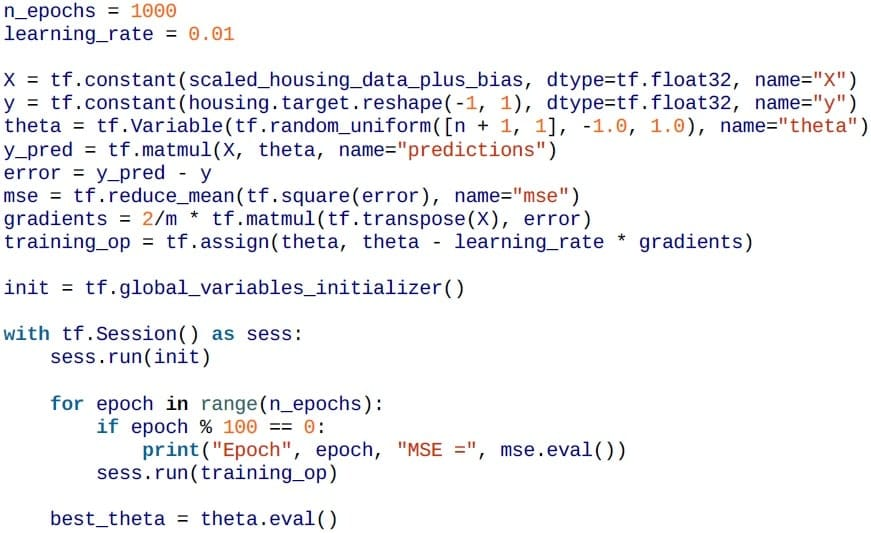Here, you compute the gradients manually.

This code assumes that normalization has been done previously.

The random_uniform function creates a random array for theta.

The weight (theta) adjustment logic is:

θ(next step) = θ -η∇θMSE(θ)

The gradient ∇θMSE(θ) is equivalent to:

(2/m)*(y_pred-y)*XT

The main loop executes the training step over and over again (n_epochs times), and at every 100 iterations it prints out the current Mean Squared Error (MSE).

One should see the MSE go down at every iteration.

In the next section, let us focus on the important features and libraries of TensorFlow.

## Important Features and Libraries

Some of the important libraries are discussed below:

tf.contrib.learn

tf.contrib.learn is a high-level TensorFlow library that simplifies the mechanics of machine learning including:

• running training loops

• running evaluation loops

• managing data sets

• managing feeding

• tf.contrib.learn defines many common models.

Autodiff

Calculating gradients manually works for Linear Regression, but for neural networks, it can be tedious.

TensorFlow has autodiff feature to automatically compute gradients.

Simply replace the gradients = … line in the previous block of code with:

Here the gradients node will compute the gradient vector of the MSE with regard to theta.

Optimizers

The optimizers of TensorFlow provide variations on Gradient Descent.

Optimizer APIs from TensorFlow abstract out the complexity of defining many of the training concepts with low-level code.

The optimizer base class provides methods to compute gradients for a loss and apply gradients to variables.

A collection of subclasses implement classic optimization algorithms such as Gradient Descent.

The Optimizer class itself need not be instantiated, but subclasses have to be.

The base class for optimizers is:

class tf.train.Optimizer

This class defines the API to add Ops to train a model.

You need not use this class directly, but instead, instantiate one of its subclasses such as GradientDescentOptimizer.

To use optimizers, replace the gradients = … and training_op = … lines in the previous block of code with:

training_op = optimizer.minimize(mse)

Or with a different Optimizer as in:

optimizer = tf.train.MomentumOptimizer(learning_rate=learning_rate, momentum=0.9)

In the next section, let us study the mini-batch gradient descent in TensorFlow.

## Mini-Batch Gradient Descent in TensorFlow

Implementation

Here, you use mini-batch gradient descent for the same problem.

For Mini-batch Gradient Descent, we need a way to replace X and Y at every iteration with the next mini-batch.

The simplest way to do this is to use placeholder nodes.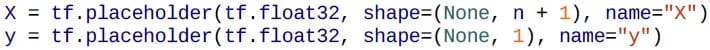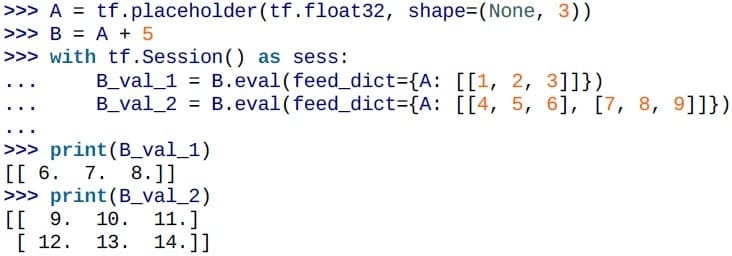Execution

To implement mini-batch gradient descent, define the batch size and the total number of batches:

batch_size = 100

n_batches = int(np.ceil(m / batch_size))

In the execution phase, fetch the batches one by one and use them to run the training operations: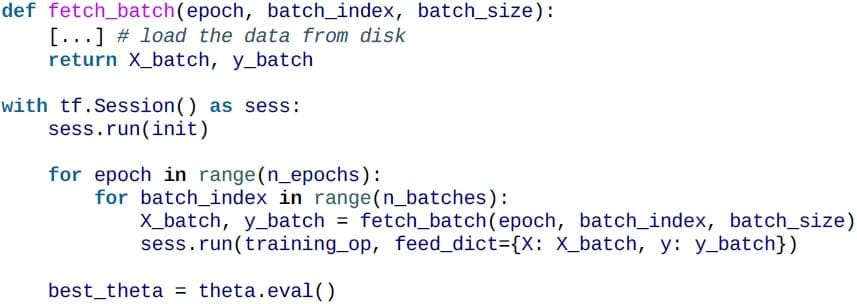Saving and Restoring Models

A trained model can be saved to disk or restored.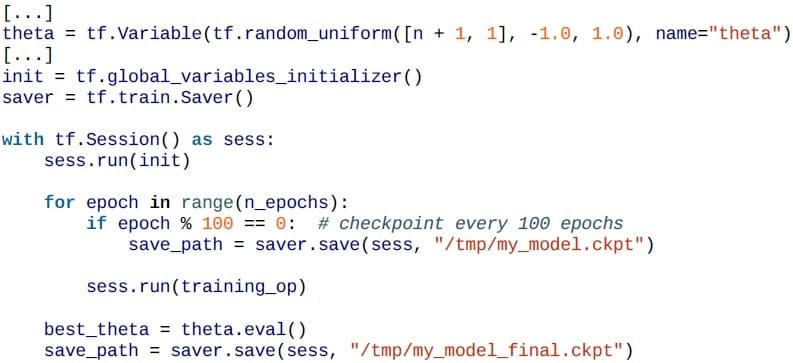Checkpoints can be stored as well in long training cycles.

Saving happens via a Saver node. During execution, call save() on the Saver node to actually save the model.

Restore happens with a call to restore function:

with tf.Session( ) as sess:

saver.restore(sess, “/tmp/my_model_final.ckpt”)

[...]

In case one wants to save only certain variables under new names:

saver = tf.train.Saver({“weights”: theta})

## TensorFlow APIs –Layers and Keras

Two Main APIs that TensorFlow provides are:

1. TensorFlow Core API - Low-level Machine Learning development

2. Higher Level APIs - More compact APIs such as tf.contrib.learn or tf.layers

Let us discuss the Higher Level APIs in the next section.

You too can join the high earners’ club. Enroll in our Deep Learning Course and earn more today.

## Higher Level APIs

Higher level APIs are built on top of TensorFlow Core. These are easier to learn and use than TensorFlow Core. They make repetitive tasks easier and more consistent between different users.

Higher level API such as tf.contrib.learn helps you manage data sets, estimators, training and inference.

Development of neural network programs can be simplified with two of the high-level TensorFlow APIs:

1. Keras API (tensorflow.contrib.keras)

2. Layers API (tensorflow.layers or tf.layers)

In the next section, let us learn how to build neural networks using layers.

## Using Layers to Build Neural Networks

You can define neural networks layer with tf.layersAPI with a simple code as given in the next section.

After defining the neural layers graph with tf.layers, the logic to define the cost function and optimizer like GradientDescentOptimizer needs to follow which is same as a normal neural network model.

After which the model training session can be executed with mini batches of training data. Later, you can test the accuracy of the model with predictions on the test data. Thus, TF.layers can prove to be a high-level TensorFlow API to simplify the neural network development via simpler code.

Example:

h1 = tf.layers.dense(inputs=tf_x, units=50,

activation=tf.tanh,

name='layer1')

h2 = tf.layers.dense(inputs=h1, units=50,

activation=tf.tanh,

name='layer2')

#fully connected third layer

logits = tf.layers.dense(inputs=h2,

units=10,

activation=None,

name='layer3')

#define predictions object for predicting the final output for test data later

predictions = {

'classes' : tf.argmax(logits, axis=1,

name='predicted_classes'),

'probabilities' : tf.nn.softmax(logits,

name='softmax_tensor')

}

This code creates a four layer neural network using tf.layers API.

Initially, two hidden layers use tanh activation function.

The third layer is a fully connected layer with 10 outputs.

The fourth layer is the Softmax layer to predict one of the 10 classes.

Let us learn how to build Neural Networks using Keras.

## Using Keras to Build Neural Networks

Keras is a high-level API used to simplify the neural network development via a simple code. It is often used as a frontend with a TensorFlow backend. Keras can be installed as a separate package.

However, after the release of TensorFlow 1.1.0, Keras has been added to the TensorFlow contrib sub-module.

Example

For the MNIST handwritten digit classification, you can classify the digit image to one of the 10 classes from zero to nine.

This data is then converted into one hot vector, which is a way to convert categorical data into numerical data as shown in the bottom of the code.

First, you need to convert the class labels (integers 0-9) into the one hot format.

y_train_onehot = keras.utils.to_categorical(y_train)

print('First 3 labels: ', y_train[:3])

print('\nFirst 3 labels (one-hot):\n', y_train_onehot[:3])

The output of this code would be:

First 3 labels:  [5 0 4]

First 3 labels (one-hot):

[[ 0.  0. 0. 0.  0. 1. 0. 0.  0. 0.]

[ 1.  0. 0. 0. 0. 0.  0. 0. 0. 0.]

[ 0.  0. 0. 0. 1. 0.  0. 0. 0. 0.]]

Example

One can define neural networks layer with tensorflow.contrib.keras API with simple code like this:

model = keras.models.Sequential()

units=50,

input_dim=X_train_centered.shape,

kernel_initializer='glorot_uniform',

bias_initializer='zeros',

activation='tanh'))

units=50,

input_dim=50,

kernel_initializer='glorot_uniform',

bias_initializer='zeros',

activation='tanh'))

units=y_train_onehot.shape,         #10 units corresponding to 10 class labels

input_dim=50,

kernel_initializer='glorot_uniform',

bias_initializer='zeros',

activation='softmax'))

This code defines the three layers of a neural network using Keras API for MNIST digit classification problem.

The third layer is a classification layer to classify the digit image to one of the 10 classes.

As seen in the code given on the previous slide, you can initialize a new model using the sequential class of Keras to implement a feedforward neural network.

Then, you can add as many layers to it as we like.

We used a new initialization algorithm for weight matrices by setting kernel_initializer='glorot_uniform’.

Glorot initialization, also known as Xavier initialization is a more robust way of initialization for deep neural networks.

Example

Compile the model with SGD optimizer and cross-entropy loss function:

sgd_optimizer = keras.optimizers.SGD(

lr=0.001, decay=1e-7, momentum=.9)

model.compile(optimizer=sgd_optimizer,

loss='categorical_crossentropy')

You now have set values for the weight decay constant and momentum learning to adjust the learning rate at each epoch.

Decay the weights and moderate the learning rate with subsequent training epochs to prevent vanishing gradients.

The binary cross-entropy is just a technical term for the cost function in the logistic regression, and the categorical cross-entropy is its generalization for multiclass predictions via softmax.

Later, run model.fit to train the model:

history = model.fit(X_train_centered, y_train_onehot,

batch_size=64, epochs=50,

verbose=1,

validation_split=0.1)

The line verbose=1 prints the optimization process over subsequent epochs.

The validation_split=0.1 reserves 10% of the training data for validation after each epoch.

Output

The output looks like this:

Train on 54000 samples, validate on 6000 samples

Epoch 1/50

54000/54000 [==============================] -3s -loss: 0.7247 -

val_loss: 0.3616

Epoch 2/50

54000/54000 [==============================] -3s -loss: 0.3718 -

val_loss: 0.2815

Epoch 3/50

54000/54000 [==============================] -3s -loss: 0.3087 -

val_loss: 0.2447

[…]

Epoch 50/50

54000/54000 [==============================] -3s -loss: 0.0485 -

val_loss: 0.1174

Let us understand what is TensorBoard in the next section.

## What Is TensorBoard?

TensorBoard allows one to understand, debug, and optimize TensorFlow programs. TensorBoard operates by reading TensorFlow event files.

Serialized data is entered in these event files when one annotates the nodes of TensorFlow with “summary operations”.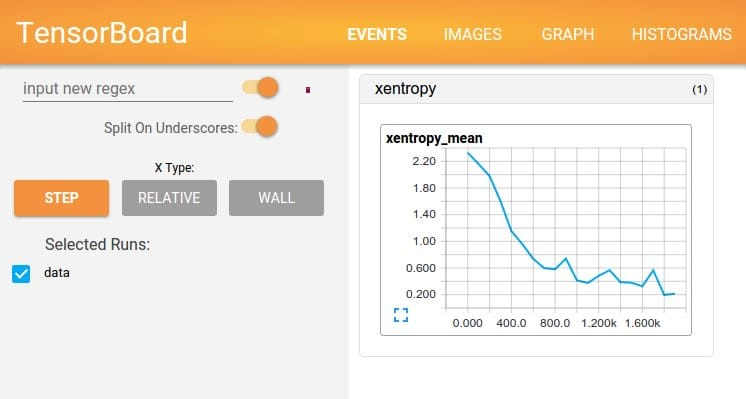This tool allows:

• Visualization of TensorFlow graph

• Plotting quantitative metrics about the execution of the graph

• Showing additional data like images that pass through it

In the next section, let us learn the steps to use TensorBoard.

## Steps to Use TensorBoard

The following steps explain how to use TensorBoard:

1. Attach the “tf.summary.scalar” ops to nodes whose output you wish to serialize. Each such scalar_summary is given a tag like “learning rate” or “loss function.”

2. Attach the “tf.summary.histogram” ops to the relevant nodes to visualize distributions of values

3. Use “tf.summary.merge_all” to merge all summary nodes ops

4. Run this to generate “Summary” protobuf object containing all summary

5. Write this object to disk using “tf.summary.FileWriter”

6. Enter “tensorboard --logdir=/tmp/tensorflow/mnist”on command prompt to start TensorBoard

7. TensorBoard is available at URL:localhost:6006

## Using Timestamp in TensorBoard

TensorBoard is an interactive visualization tool that shows learning curves and lots of other plots in the web browser.

It requires the code to log the stats and graph details to a log directory. One needs to use a different log directory in each run of the program, else log data from multiple runs will get merged and mess up TensorBoard.

The simplest solution for this is to include a timestamp in the log directory name.

from datetime import datetime

now = datetime.utcnow( ).strftime(“%Y%m%d%H%M%S)

root_logdir = “tf_logs”

logdir = “{ }/run-{ }/”.format(root_logdir, now)

Add the following code at end of construction phase:

mse_summary = tf.summary.scalar(‘MSE’, mse)

file_writer = tf.summary.FileWriter(logdir, tf.get_default_graph())

In the next section, let us focus on Visualization using TensorBoard.

## Visualization with TensorBoard

TensorBoard helps to understand the learning process and its results.

Next, update the execution phase to evaluate the mse_summary node regularly during training (For example, every 10 mini batches).

This will output a summary that one can then write to the events file using the file_writer.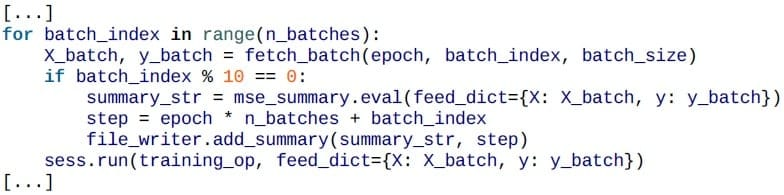Finally, close the file writer at the end of the program.

file_writer.close()

Check the working directory: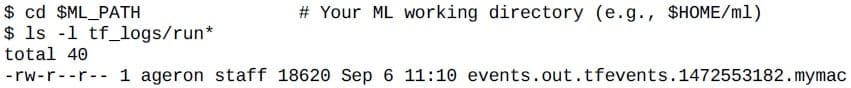After a second run: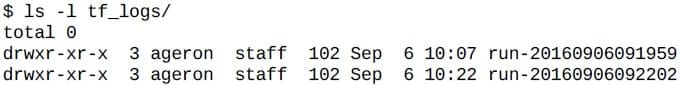Now start the TensorBoard server: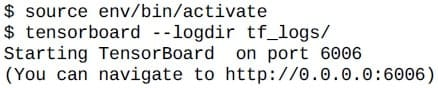Next, open a browser and go to http://0.0.0.0:6006/ (or http://localhost:6006/)

Let us look at the events tab on TensorBoard in the next section.

## Events Tab on TensorBoard

TensorBoard shows a lot of useful information on MSE, gradients, computation graph, variances, standard deviation etc.

In the chart given below, you can see the MSE and how it declines over subsequent Epochs: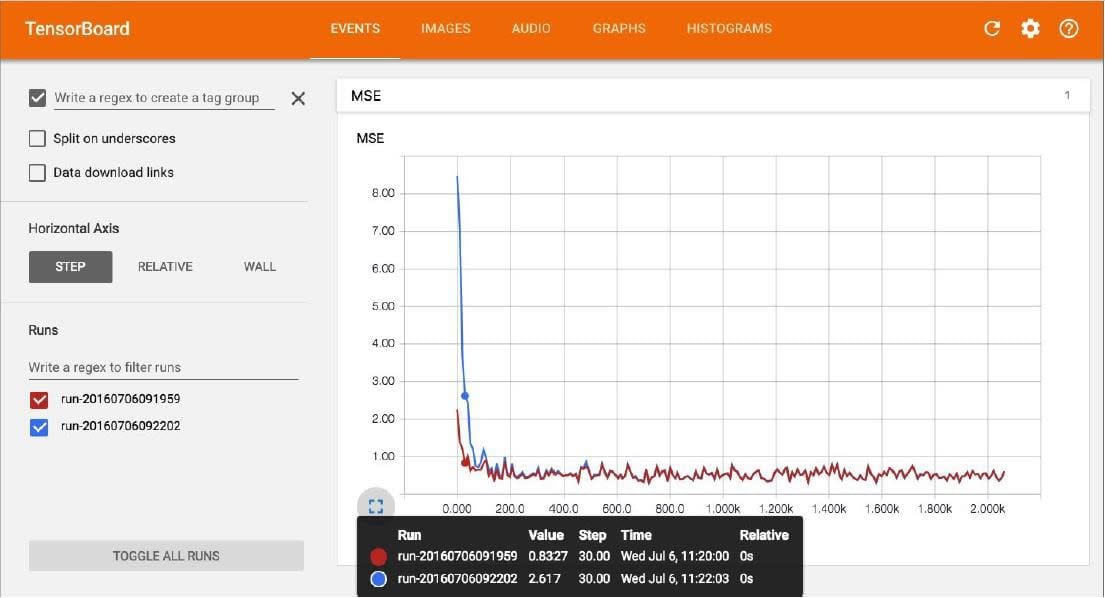The blue and red line shows two different instances of the program being run.

Let us look at the graphs tab on TensorBoard in the next section.

## Graphs Tab on TensorBoard

TensorBoard Graphs section shows the actual computation graph built by TensorFlow for the program.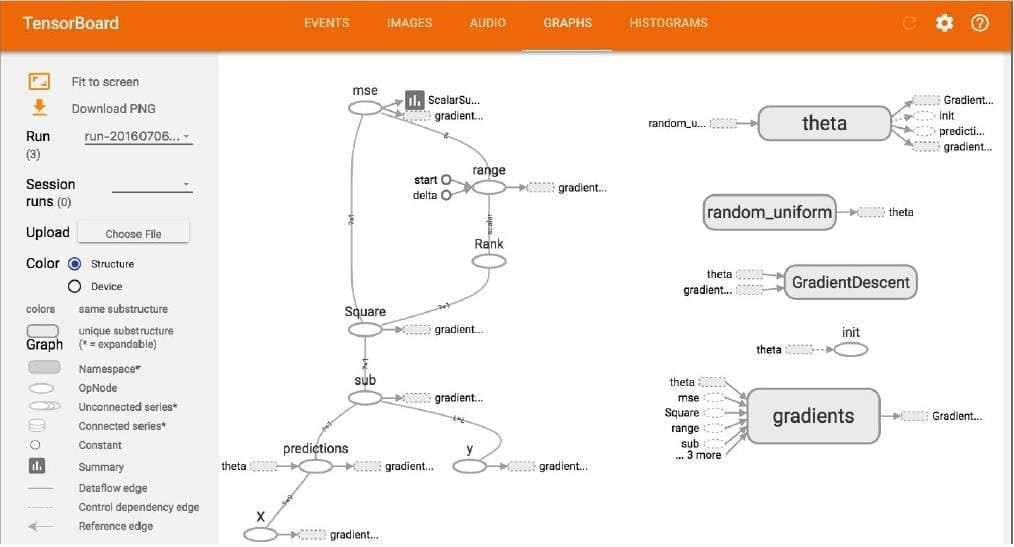## Summary

Let us summarize what we have learned in this lesson:

• TensorFlow is one of the most popular Deep Learning libraries.

• It allows parallel computation over high-performance GPU processors.

• It works by first creating a Computation Graph of the program logic before the actual execution is done.

• TensorFlow Core API allows low-level programming for machine learning.

• Keras and TF.Layers are higher level APIs in TensorFlow, which make the development of neural networks simpler.

• Trained models can be serialized to disk and restored again in code.

• TensorFlow includes TensorBoard, a powerful visualization tool to understand the machine learning process.

## Conclusion

This concludes the lesson “Introduction to TensorFlow.” The next lesson is “Training Deep Neural Networks.”

Related Courses
Learner Reviews
Related Articles
• Disclaimer
• PMP, PMI, PMBOK, CAPM, PgMP, PfMP, ACP, PBA, RMP, SP, and OPM3 are registered marks of the Project Management Institute, Inc.

Name*
Email*
Phone Number*